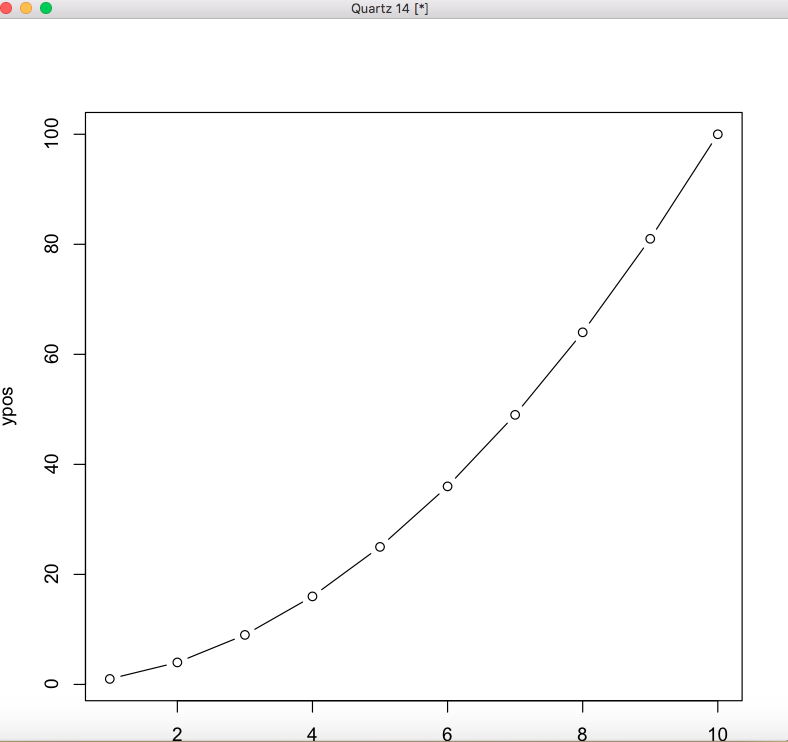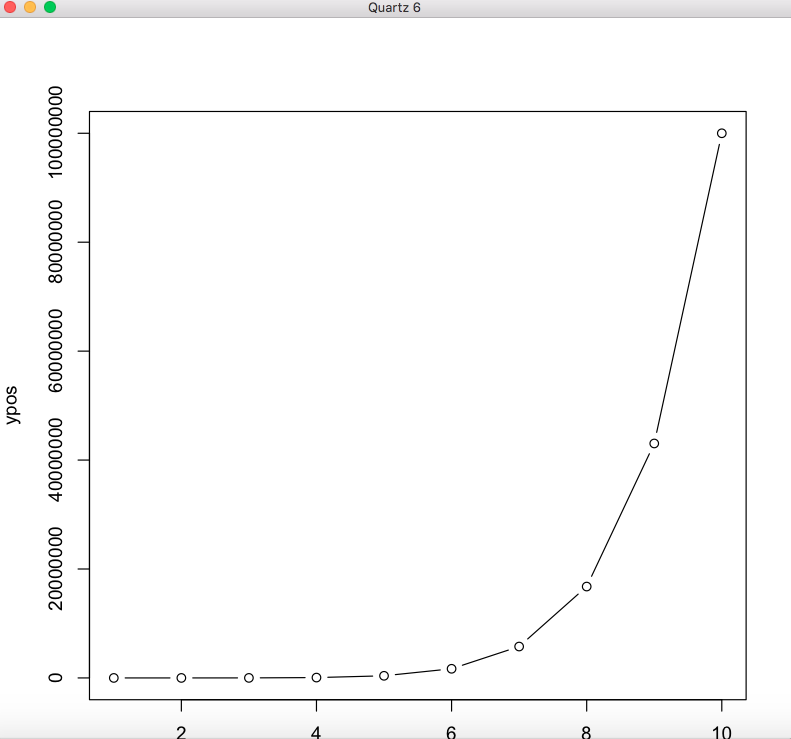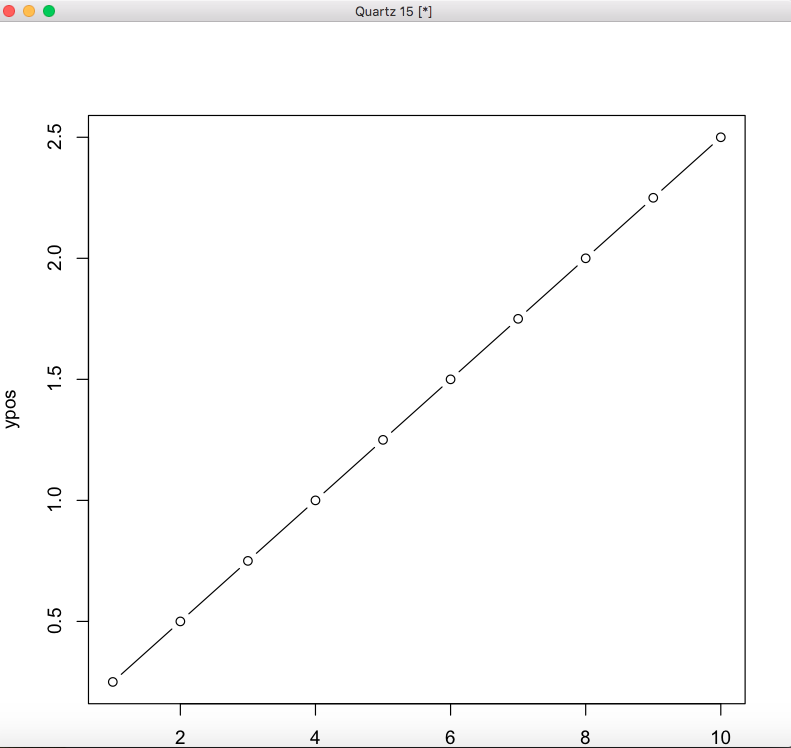Related Articles

# Create Plot Window of Particular Size in R

• Last Updated : 16 May, 2021

In this article, we will discuss various methods available in the R programming language to adjust the plot window size.

### Method 1 : Using dev.new() method

The width and height of the plot can be specified along with the units to display the plot in. The usage of this method can be customized to fit the graph well within the plot window size.

Syntax:

dev.new(width, height, unit = c(in, px, cm))

Example 1: Specifying the size in inches

## R

 `# declaring the xpos vector ``xpos <- ``c``(1:10)`` ` `# declaring the ypos vector equivalent ``# to x^2``ypos <- xpos^2`` ` `# specifying the dimensions of the plot ``# window in inches``dev.new``(width=20, height=10, unit=``"in"``)`` ` `# plotting the points in plot window``plot``(xpos,ypos, type=``"b"``)`

OutputThe dimensions of height and width are larger in this case.

Example 2: Specifying the size in pixels

## R

 `# declaring the xpos vector ``xpos <- ``c``(1:10)`` ` `# declaring the ypos vector equivalent ``# to x^2``ypos <- xpos^8`` ` `# specifying the dimensions of the plot ``# window in inches``dev.new``(width=300, height=150, unit=``"px"``)`` ` `# plotting the points in plot window``plot``(xpos,ypos, type=``"b"``)`

Output### Method 2 : Using windows.options method

windows.options() method is only available in Windows machines. Width and height arguments can be specified to the method to override the default settings and according to these settings are applied till the settings are refreshed using factory.reset().

Syntax:

windows.options(width, height, reset = FALSE)

Example:

## R

 `# declaring the xpos vector ``xpos <- ``c``(1:10)`` ` `# declaring the ypos vector equivalent``# to x^2``ypos <- xpos^2`` ` `# specifying the dimensions of the plot ``# window in inches``windows.options``(width = 20, height = 10, reset = ``FALSE``)`` ` `# plotting the points in plot window``plot``(xpos,ypos, type=``"b"``)`

Output### Method 3: Using noRStudioGD option

noRStudioGD = TRUE is an optional parameter in R that can be used to draw plots in earlier versions of R. It can be set in the dev.new() method, in case the method fails to open a device driver.

Syntax:

dev.new(width, height, unit = c(in, px, cm),noRStudioGD = TRUE)

Example:

## R

 `# declaring the xpos vector ``xpos <- ``c``(1:10)`` ` `# declaring the ypos vector equivalent ``# to x^2``ypos <- xpos^1/4``  ` `# specifying the dimensions of the plot ``# window in inches``dev.new``(width=50, height=25, unit=``"cm"``,noRStudioGD = ``TRUE``)`` ` `# plotting the points in plot window``plot``(xpos,ypos, type=``"b"``)`

OutputMy Personal Notes arrow_drop_up